﻿ Heat Radiation by Forced Convection(Transient Analysis)Examples | Product | Murata Software Co., Ltd.# Example8Heat Radiation by Forced Convection(Transient Analysis)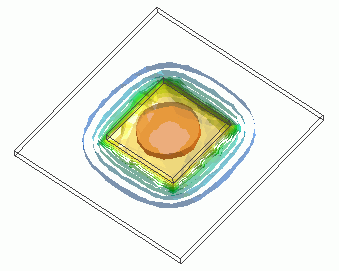General

• The model is the same as Exercise 7: A heat source is placed on a substrate, and there is a forced air flow for cooling in parallel to the substrate. The heat radiation is analyzed under the transient condition.

• The heat transfer coefficient is acquired manually.
To acquire it automatically, see “Ex.1 of Simple Fluid-Thermal Analysis”.

• The temperature distribution and the heat flux vectors are solved.

• Unless specified in the list below, the default conditions will be applied.

### Analysis Space

 Item Settings Analysis Space 3D Model unit mm

### Analysis Conditions

 Item Settings Solver Thermal Analysis [Watt] Analysis Type Transient analysis Options N/A

The transient analysis is set up as follows. The total number of steps is 20. The time step is 30 second.

Therefore, the temperature distributions for 600 seconds are solved.

Tabs

Setting Item

Settings

Transient analysis

Table

 Number Calculation steps Output steps Time step [s] 1 20 1 30

Initial Temperature

25[deg]

### Model

The same as Exercise 7. The material properties and the boundary conditions are the same as well.

The substrate (VOL1) and the heat source (VOL2) are created as solid body box, and the heat amount is defined in the body attribute of VOL2.

The heat transfer coefficients for the top and bottom faces of the substrate and the top face of the heat source are calculated basede on the simplified equation.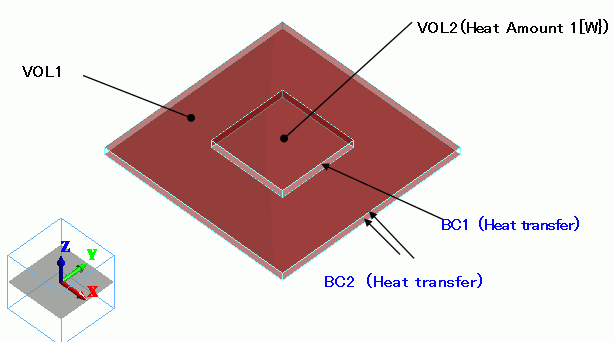### Body Attributes and Materials

 Body Number/Type Body Attribute Name Material Name 0/Solid VOL1 006_Glass_epoxy * 1/Solid VOL2 001_Alumina *

* Available from the Material DB

The heat source of VOL2 is set up as follows.

 Body Attribute Name Tab Settings VOL2 Heat Source 1[W]

### Boundary Conditions

The heat transfer coefficients for the forced convection are calculated as follows. For the details, please refer to the Heat Transfer Coefficient for Forced Convection

To acquire it automatically, see “Ex.1 of Simple Fluid-Thermal Analysis”.

h = 3.86 x (V/L)0.5xC [W/m2/deg]

where

Air flow V=1[m/s]

On the top and bottom faces of VOL1: Characteristic length L=0.05, C=1 -> h=17.26
Top face of the heat source (VOL2): Characteristic length L=0.02, L’=0.015, C=1 * -> h=27.3

*

The thickness (d) of the speed boundary layer at the edges of the heat source is calculated as follows

d=0.0182x(L’/V)0.5= 2.3[mm]

This is close enough to the thickness of heat source, so we set C=1.

 Boundary Condition Name/Topology Tab Boundary Condition Type Settings BC1/Face Thermal Heat Transfer/Ambient Radiation Heat transfer coefficient: 17.26 [W/m2/deg] Ambient temperature: 25[deg] BC2/Face Thermal Heat Transfer/Ambient Radiation Heat transfer coefficient: 27.3 [W/m2/deg] Ambient temperature: 25[deg]

### Results

The temperature distributions at each time are shown below.

At Minimum/Maximum Value on the Contour tab of [Graphics Setup], deselect “Automatic” and set 25 => 62.

##### In 60 seconds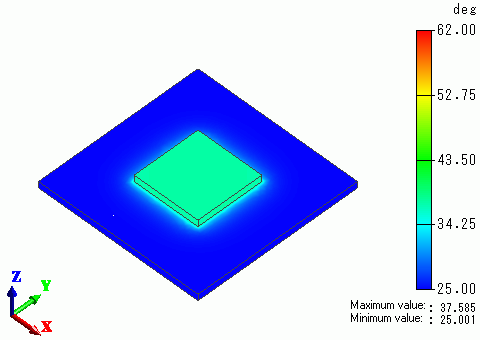##### In 180 seconds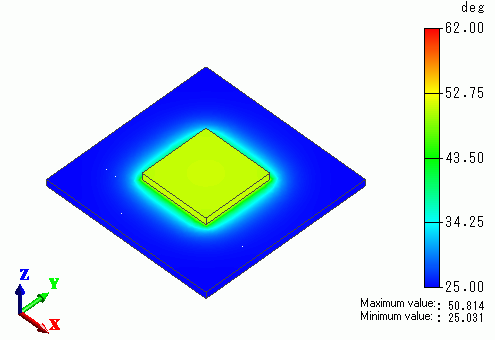##### In 360 seconds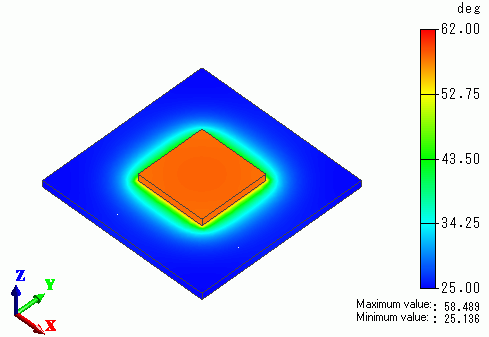The temperature vs. time is plotted for the center of the heat source.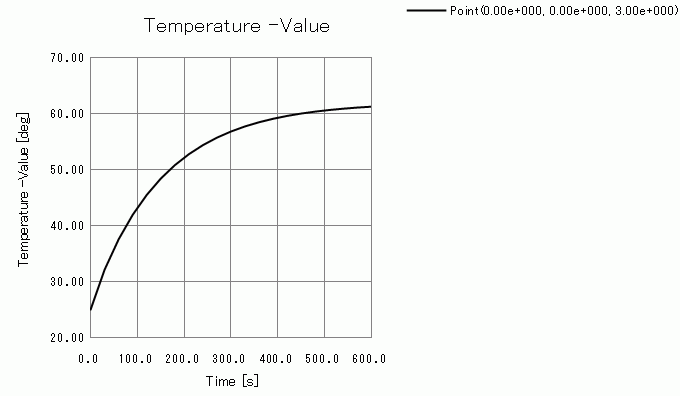It is getting stabilized in 600 seconds.

﻿